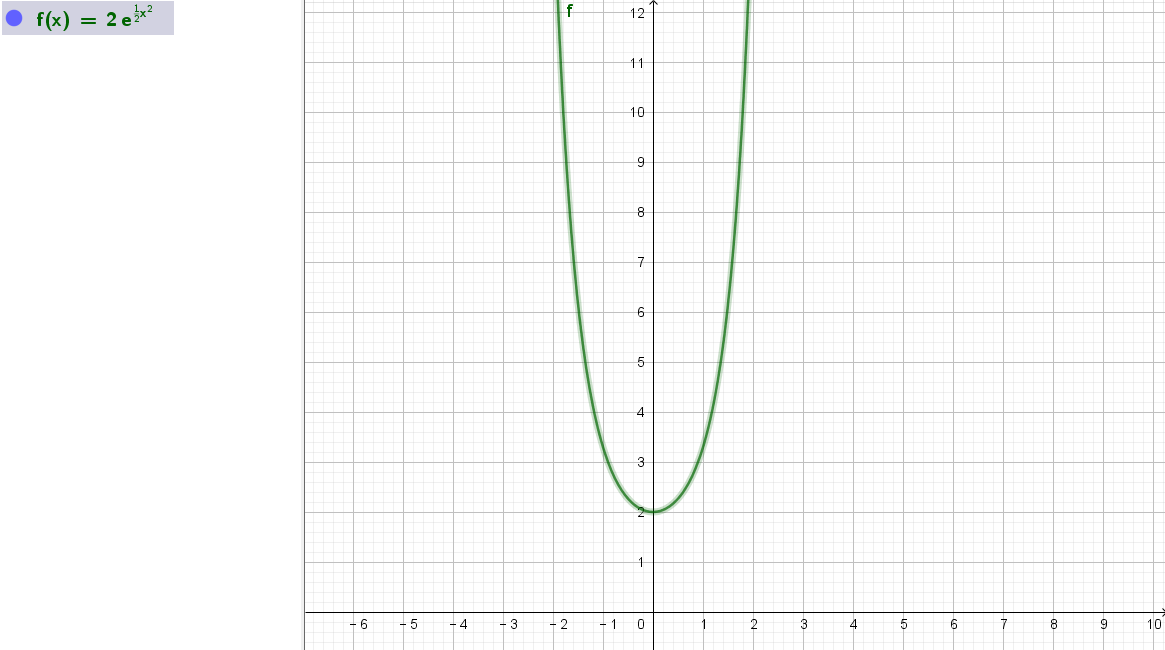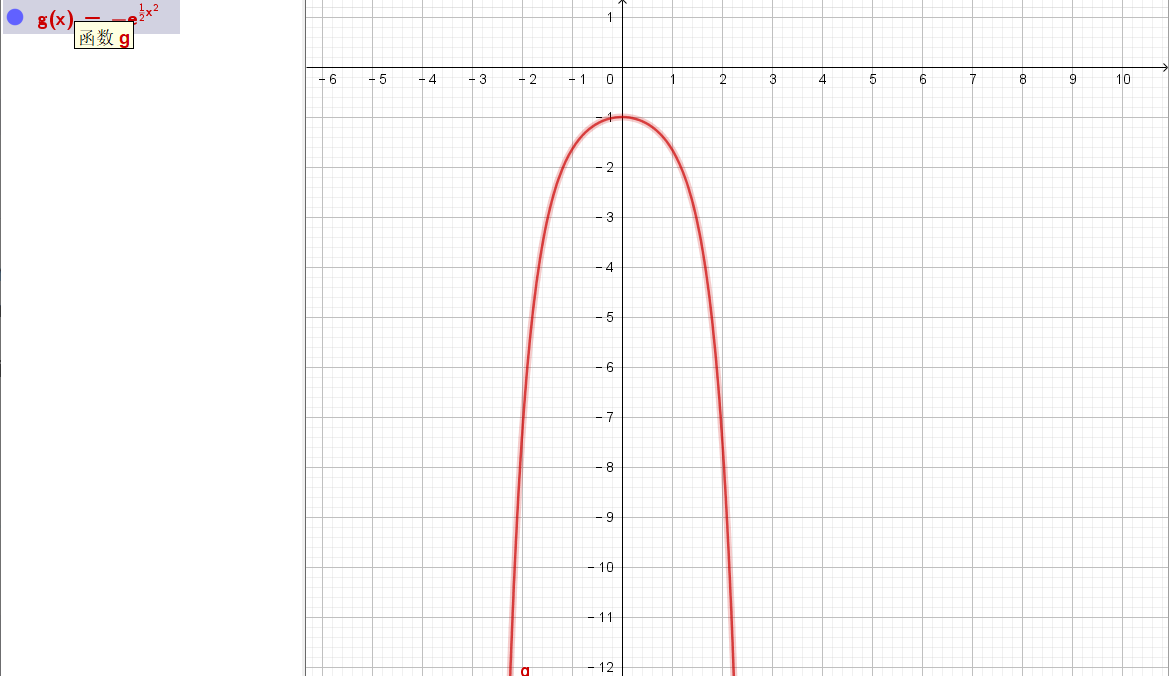### ¿Todavía tienes preguntas de matemáticas?

Pregunte a nuestros tutores expertos
Algebra
Pregunta

19. The calculator drawn slope field for the differential equation $$\frac { d y } { d x } = x y$$ is shown in the figure below. The solution curve passing through the point $$( 0,1 )$$ is also shown. (a) Sketch the solution curve through the point $$( 0,2 )$$ . (b) Sketch the solution curve through the point $$( 0 , - 1 )$$ .